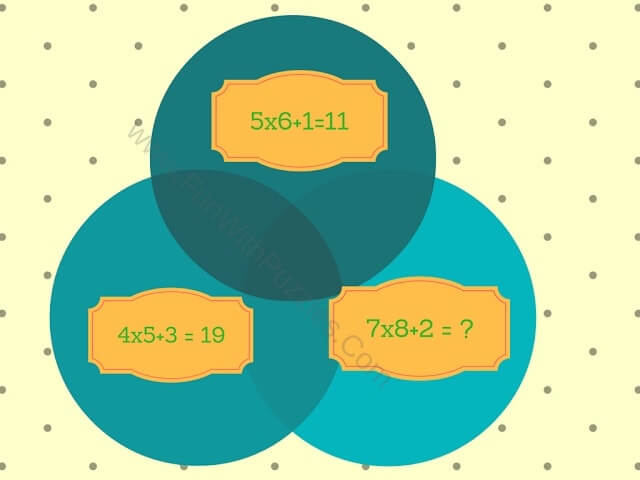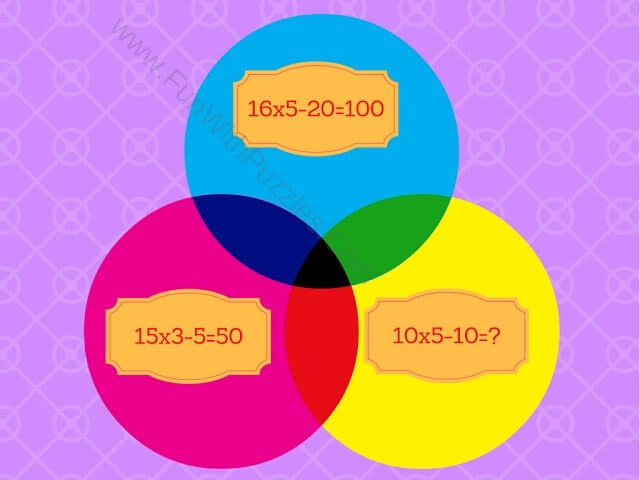In all these puzzles, three circles are given with the mathematical equations. At first glance, these math equations look incorrect. However, if we replace some or all of the given mathematical operation with other math operation, then these equations become correct. Study the given two equations in the circles to find which operation can be replaced and then apply it on the third circle to solve the given mathematical equation.
Please do note that BODMAS rules follow while solving the mathematical puzzles.
Answers link to these Mathematical Equations picture puzzles is given at the end of this post.Mathematics Equations Circle Picture Puzzle-1Mathematics Equations Circle Picture Puzzle-2Mathematics Equations Circle Picture Puzzle-3Mathematics Equations Circle Picture Puzzle-4Mathematics Equations Circle Picture Puzzle-5